Source Paper: Easy Volatility Investing

Tony Cooper, Double Digit Numerics

Feb 2013

Abstract

Historically volatility trading are only available through options, OTC variance swaps, and later VIX futures.

Volatility ETPs are making volatility trading accessible to retail investors and fund managers.

This purpose of this paper is to devise trading strategies using volatility ETPs.

This paper is structured in following topics:

• Where volaitlity returns come from
• Portfolio diversification with volatiltiy as an asset class

1. Introduction

VIX Stylized Facts:

• VIX, unlike stock market returns, is predictable
• Changes in VIX are negatively correlated with changes in market returns
• Investors pay Volatiltiy Risk Premium to hedge their volatility risks

Paper Structure:

• Volatility Risk Premium (VRP) provides our volatility returns, not Roll Yield
• Volatilty ETPs: VXX/XIV
• Strategies
• Risks:
• Volatility Drag
• Timing Synchronization
• VRP-Roll Yield Convergence
• Regime Change Risk
• Steamrollr Risk
• Diversification using volatility ETPs
• Low correlation to S&P500
• Boost Returns
• Lower Volatilities
• Reduce Drawdowns
• Improve Sharpe

2. The Lure and Intrigue of Volatiltiy

CBOE VIX

VIX is predictable - It tends to mean revert

• Simple mean-reverting strategy based on 11-day moving average (long VIX when below MA-11, else go short) provides 215% annualized returns

Changes in VIX is negative correlated with changes in S&P500 (refer to diagram below, notice the correlation is becoming more negative)

• Long position in VIX, provided it generates positive returns, would provide portfolio diversification

Diagram below shows rolling 1-year correlation between daily SPX return and VIX return.

In :
import pandas as pd
import numpy as np
import matplotlib.pyplot as plt
%matplotlib inline

data = pd.DataFrame()
data['VIX_Ret'] = data['VIX'].pct_change()
data['SPX_Ret'] = data['SPX'].pct_change()
data['VIX_Ret'].rolling(252).corr(data['SPX_Ret']).plot(figsize=(15,4))

Out:
<matplotlib.axes._subplots.AxesSubplot at 0x122cc5950>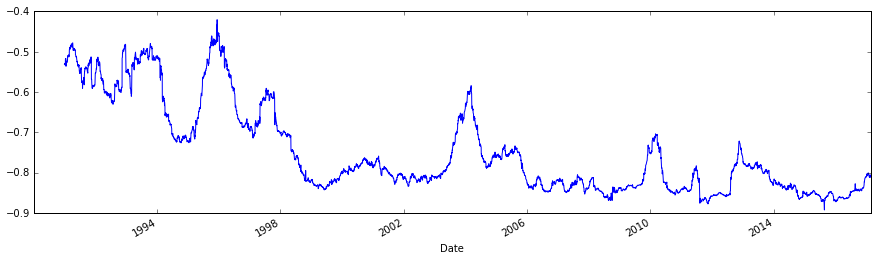Clearly, the above graph shows the correlation is consistently negative and it is trending toward more negative

Take a look for VIX vs. XIV performance duing 2012-04-03 and 2012-10-01 period:

In :
data_scaled = pd.DataFrame()
data_scaled['VIX'] = data['VIX']/data.ix['2012-04-03']['VIX']
data_scaled['XIV'] = data['XIV']/data.ix['2012-04-03']['XIV']
data_scaled.ix['2012-04-01':'2012-10-01'][['VIX','XIV']].plot(figsize=(15,8))

Out:
<matplotlib.axes._subplots.AxesSubplot at 0x122ccfcd0>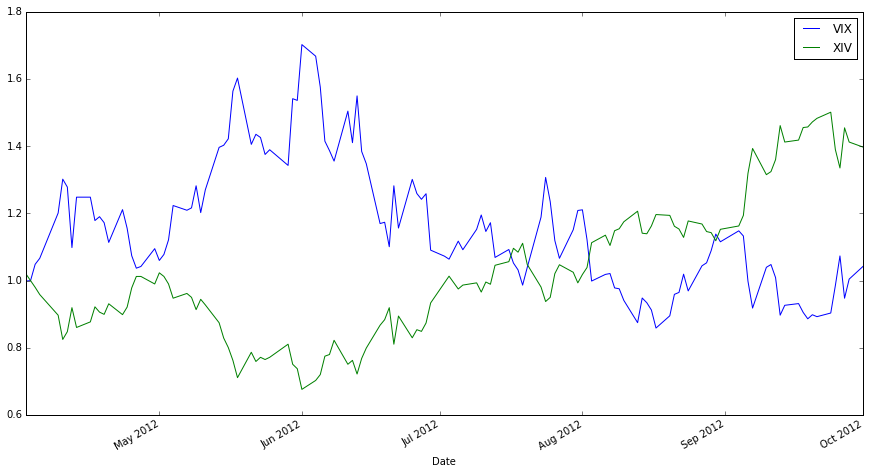The above graph shows that during the period, while VIX finishes almost flat, XIV is 40% up

VRP - Volatility hedgers prepared to pay volatility speculators to offload volatility risk

• It represents the amount that VIX overpredicts S&P500 volatility
• The following diagram illustrates spot VIX vs. rolling realized historical volatility 30 days after (using 21 trade days)
• It's obvious that VIX dominates 30-day realized volaitlity (after shift forward for 30 days) most of the time
• This represents the VRP is positive most of the time
In :
data['SPX_HV21'] = data['SPX_Ret'].rolling(21).std() * np.sqrt(252) * 100
data['SPX_HV21_Shift'] = data['SPX_HV21'].shift(-21)
data[['VIX','SPX_HV21_Shift']].plot(figsize=(15,8))

Out:
<matplotlib.axes._subplots.AxesSubplot at 0x122032cd0>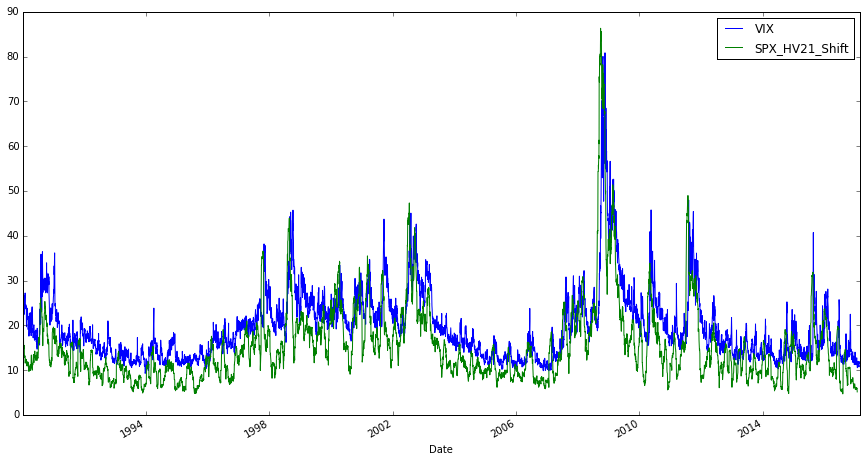Furthermore, below diagram shows the log differences between spot VIX and realized volatility 30 days after.

In :
(np.log(data['VIX']) - np.log(data['SPX_HV21_Shift'])).plot(figsize=(15,8),grid=1)

Out:
<matplotlib.axes._subplots.AxesSubplot at 0x123926190>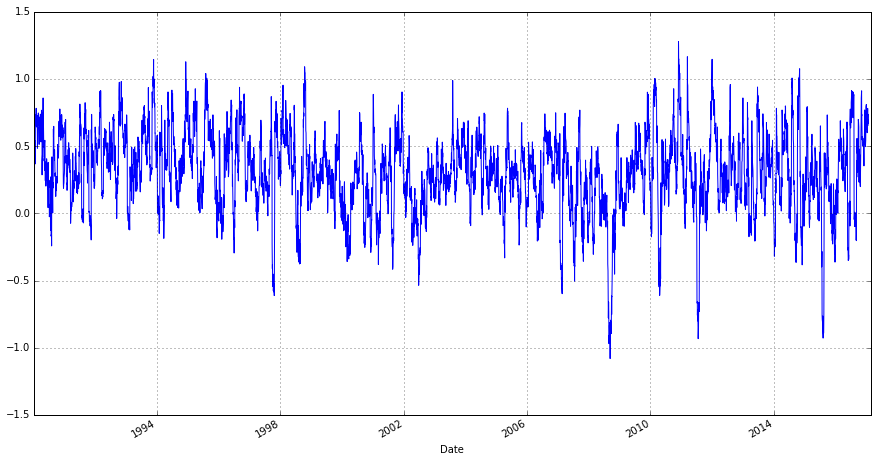4. VIX Futures

The VIX ETPs are constructed using VIX Futures. VIX futures are cash settled using VIX value on expiry/settlement day.

Since VIX Index is not directly tradable, VIX futures price reflects market collective estimate of future VIX level.

Below diagram illustrates VIX Futures Term Structure in Contango on Feb 23, 2017.

In :
import sys
import cboe_vx as cboe

VXF = pd.DataFrame()
VXF['VIX'] = data['VIX']
f = cboe.getCboeData(2017,3)
VXF['Mar'] = f['Settle']
f = cboe.getCboeData(2017,4)
VXF['Apr'] = f['Settle']
f = cboe.getCboeData(2017,5)
VXF['May'] = f['Settle']
f = cboe.getCboeData(2017,6)
VXF['Jun'] = f['Settle']
f = cboe.getCboeData(2017,7)
VXF['Jul'] = f['Settle']
f = cboe.getCboeData(2017,8)
VXF['Aug'] = f['Settle']
f = cboe.getCboeData(2017,9)
VXF['Sep'] = f['Settle']
f = cboe.getCboeData(2017,10)
VXF['Oct'] = f['Settle']
VXF.ix['2017-02-23'].plot(figsize=(15,5))

Out:
<matplotlib.axes._subplots.AxesSubplot at 0x123cb6b10>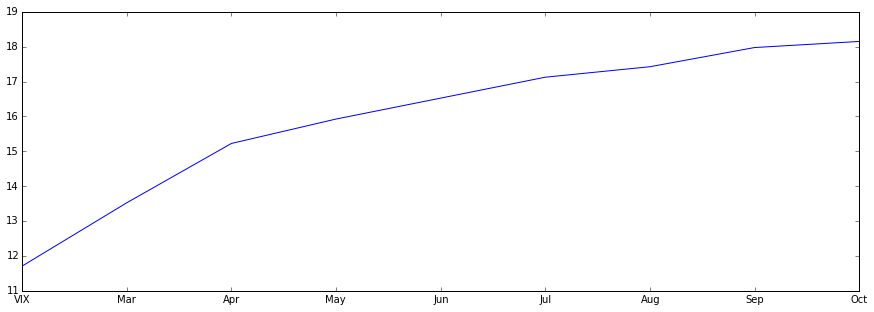Diagram below illustrates a backwardation term structure - happened on Oct 03, 2008, during Financial Crisis.

In :
VXF = pd.DataFrame()
VXF['VIX'] = data['VIX']
f = cboe.getCboeData(2008,10)
VXF['Oct'] = f['Settle']
f = cboe.getCboeData(2008,11)
VXF['Nov'] = f['Settle']
f = cboe.getCboeData(2008,12)
VXF['Dec'] = f['Settle']
f = cboe.getCboeData(2009,1)
VXF['Jan'] = f['Settle']
f = cboe.getCboeData(2009,2)
VXF['Feb'] = f['Settle']
f = cboe.getCboeData(2009,3)
VXF['Mar'] = f['Settle']
f = cboe.getCboeData(2009,4)
VXF['Apr'] = f['Settle']
f = cboe.getCboeData(2009,5)
VXF['May'] = f['Settle']
f = cboe.getCboeData(2009,6)
VXF['Jun'] = f['Settle']
VXF.ix['2008-10-03'].plot(figsize=(15,5))

Out:
<matplotlib.axes._subplots.AxesSubplot at 0x12381c0d0>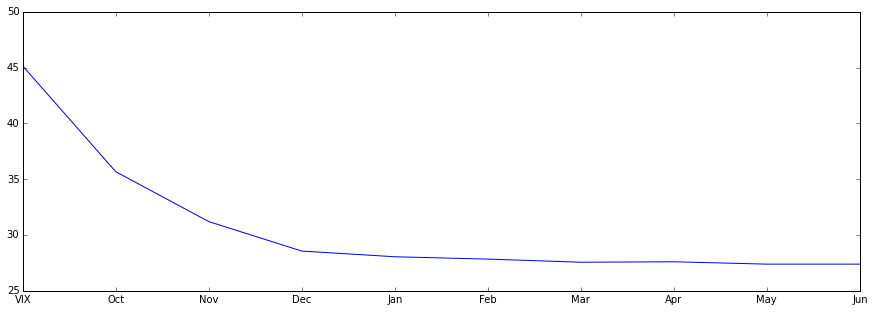Now the question is - is VRP present in VIX futures?

This can be tested using a hypothetical constant one-month future against VIX at 30 days later (when future settles).

Diagram below tries to illustrate this:

• the constant one-month future is constructed using time-weighted values from F1 and F2
• VIX is shifted forward for 30 days (21 trade days)
In :
import Quandl
VXF30 = pd.DataFrame()
x = Quandl.get("CHRIS/CBOE_VX1") # continuous F1
VXF30['F1'] = x['Settle']
x = Quandl.get("CHRIS/CBOE_VX2") # continuous F2
VXF30['F2'] = x['Settle']
calendar = pd.read_csv('f1_f2_ttm.csv') # read in expiry dates and days till maturity
calendar = calendar.set_index('Date')
VXF30 = pd.merge(VXF30, calendar, how = 'left', left_index = True, right_index = True)
VXF30['X1'] = 30 - VXF30['TTM1']
VXF30['X2'] = VXF30['TTM2'] - 30
VXF30['W1'] = VXF30['X2'] / (VXF30['X1'] + VXF30['X2'])
VXF30['W2'] = VXF30['X1'] / (VXF30['X1'] + VXF30['X2'])
VXF30['VXF30'] = VXF30['F1'] * VXF30['W1'] + VXF30['F2'] * VXF30['W2']
VXF30['VIX'] = data['VIX']
VXF30['VIX_ShiftF21'] = data['VIX'].shift(-21)
#VXF30['VIX'] = data['VIX'].shift(-21)
VXF30[['VXF30','VIX_ShiftF21']].ix['2007-10-01':].plot(figsize=(15,8))

Out:
<matplotlib.axes._subplots.AxesSubplot at 0x12389fc10>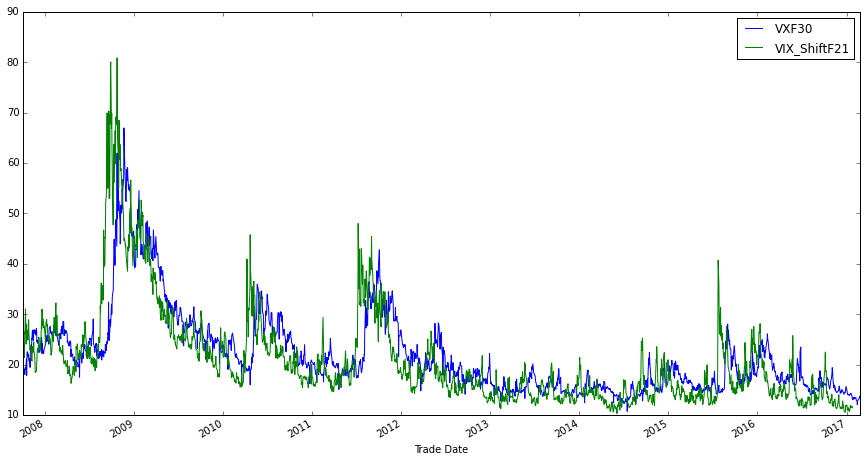As we can see, the predicted 30-day VIX future dominates subsequent 30-day VIX spot for the majority of times, i.e., Volatility Risk Premium presents in the futures market.

Diagram below shows log difference between one-month VIX futures and subsequent 30-day VIX spot.

In :
(np.log(VXF30['VXF30'].ix['2007-10-01':]) - np.log(VXF30['VIX_ShiftF21'].ix['2007-10-01':])).plot(figsize=(15,8),grid=True)
(np.log(VXF30['VXF30'].ix['2007-10-01':]) - np.log(VXF30['VIX_ShiftF21'].ix['2007-10-01':])).mean()

Out:
0.07114057198623923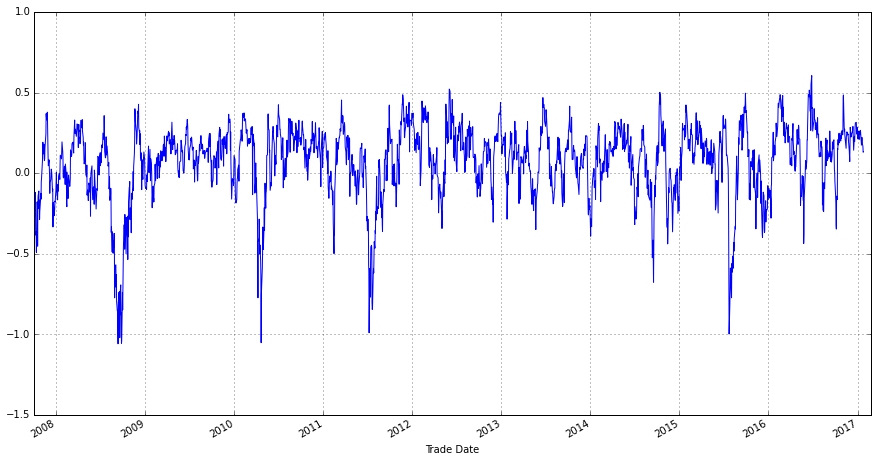5. Roll Yield

An important question in trading VIX futures is:

• does spot VIX converges to future's price, or does the future's price converges to spot VIX?

Roll Yield describes the differnce between spot VIX and future's price.

Roll yield is measurable, VRP is not.

Roll yield is positive when term structure is in Contango, negative when in Backwardation.

The roll yield is shown in the diagram below.

In :
VXF['F1_VIX_Yield'] = (VXF30['F1'] - VXF30['VIX']) / VXF30['VIX']
VXF['F1_VIX_Yield'].ix['2007-10-01':].plot(figsize=(15,8),grid = True)

Out:
<matplotlib.axes._subplots.AxesSubplot at 0x127c09d50>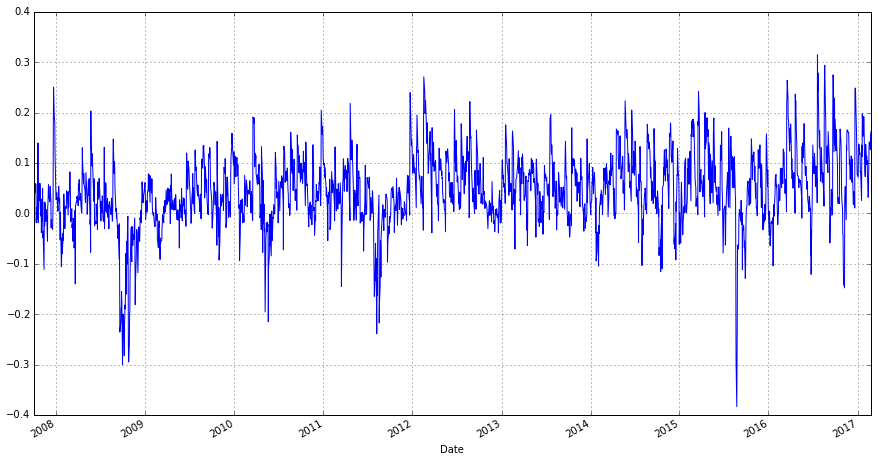And it averages at 4.3% since Oct 01, 2007.

In :
VXF['F1_VIX_Yield'].ix['2007-10-01':].mean()

Out:
0.04375067982717356

6. ETPs based on VIX Futures

Based on S&P 500 Short Term Futures: VXX/XIV

Based on S&P 500 Mid Term Futures: VXZ/ZIV

Many other ETNs including leveraged and inverse leveraged, all share similiar structure.

7. XIV Dynamics

XIV invests in short positions of VIX futures

• When futures go down, XIV goes up
• Due to the positive correlation between VIX futures and VIX spot, usually when VIX goes down, XIV goes up (not always)

XIV shifts positions between 1st and 2nd month futures to maintain CONSTANT one-month futures.

Diagram below illustrates daily XIV returns vs. VIX returns.

In :
import OLS_Regression as ols
data['XIV_Ret'] = data['XIV'].pct_change()
ols.linreg(data['VIX_Ret'].ix['2010-12-01':].values, data['XIV_Ret'].ix['2010-12-01':].values)

Out:
Dep. Variable: R-squared: y 0.777 OLS 0.777 Least Squares 5467. Mon, 27 Feb 2017 0.00 17:05:29 3985.9 1569 -7968. 1567 -7957. 1 nonrobust
coef std err t P>|t| [95.0% Conf. Int.] 0.0032 0.000 6.540 0.000 0.002 0.004 -0.4615 0.006 -73.940 0.000 -0.474 -0.449
 Omnibus: Durbin-Watson: 325.757 2.115 0 2976.43 -0.697 0 9.602 13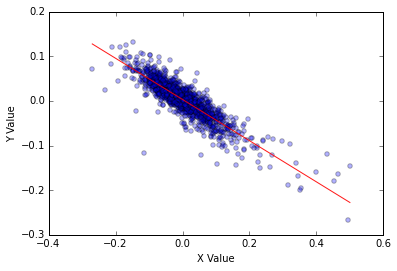Further, we define:

• XIV Expected Daily Roll Yield = (F2 - F1)/F1/30

We will show expected daily roll yield since XIV inception, and its cumulative roll yields.

In :
VXF30['F2_F1_Yield'] = (VXF30['F2'] - VXF30['F1'])/(VXF30['F1'])/30
(VXF30['F2_F1_Yield'].ix['2010-10-30':]*100).plot(figsize=(15,8), grid = True)

Out:
<matplotlib.axes._subplots.AxesSubplot at 0x129ca7090>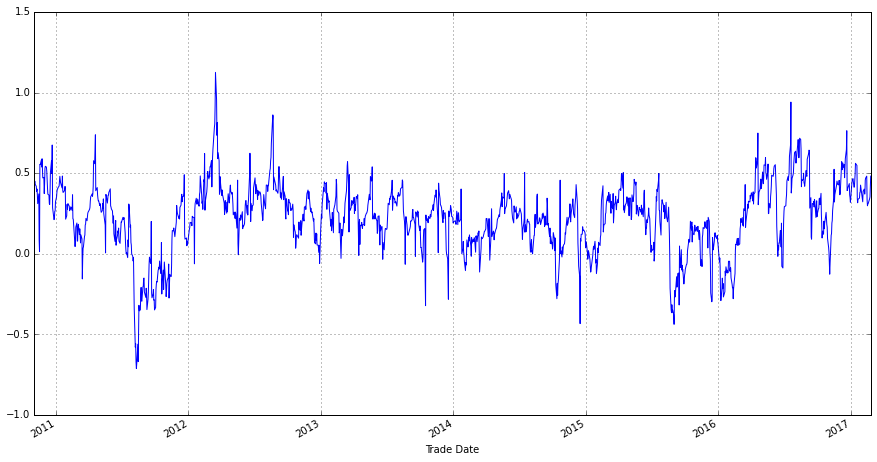Above graph also clearly shows F1~F2 contango vs. backwardation periods:

• Days above zero, F1 and F2 are in Contango
• Days below zero, F1 and F2 are in Backwardation
In :
VXF30['F2_F1_Yield'].ix['2010-10-30':].add(1).cumprod().plot(figsize=(15,8),grid=True)

Out:
<matplotlib.axes._subplots.AxesSubplot at 0x12a2aed50>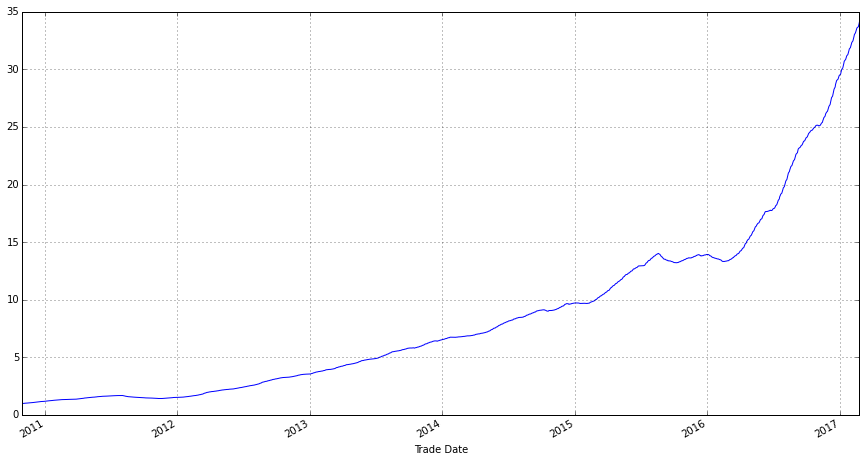The above diagram illustrates if the expected daily roll yield can be realized, XIV will harvest magnificant returns over time. Of course, it didn't happen.

8. More XIV Dynamics

Compare price performance since inception for VXX (2009-01-29) and XIV (2010-11-30).

In :
data['VXX'] = web.DataReader('vxx','yahoo','2009-01-29')['Adj Close']
data[['VXX']].ix['2009-01-29':].plot(figsize=(15,4))

Out:
<matplotlib.axes._subplots.AxesSubplot at 0x126511390>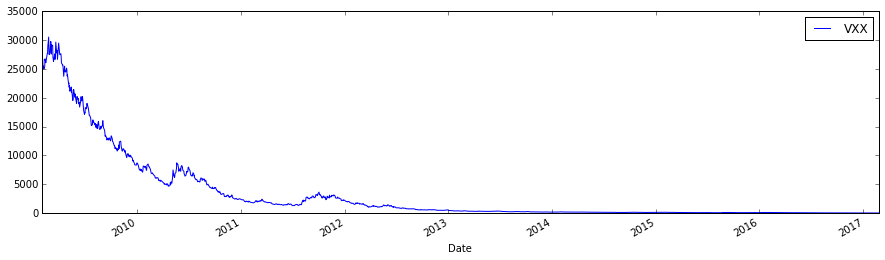In :
data[['XIV']].ix['2010-11-30':].plot(figsize=(15,4))

Out:
<matplotlib.axes._subplots.AxesSubplot at 0x1267cc1d0>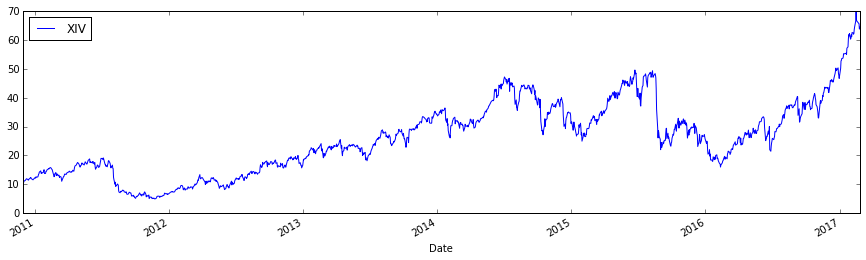Notes for above two diagrams:

• XIV is the inverse of VXX, however XIV does not have corresponding gains vs. the magnitude of VXX losses
• This is due to the compounding effect for daily inverse returns
• There is also a volatility drag that hinders the return for the leveraged and inverse ETFs
• For XIV, the biggest one-day loss of -26.8% happened on June 24, 2016
• For XIV, maximum drawdown is -74%, happened during July 07, 2011 and Nov 25, 2011; using hypothetical data going back to the start of VIX futures in 2004, the maximum drawdown would have been -93%
In :
ret = data['XIV_Ret'].add(1).cumprod()
dd = ret.div(ret.cummax()).sub(1)
mdd = dd.min()
end = dd.argmin()
start = ret.loc[:end].argmax()
print "Maximum Drawdown and Period: ", mdd, start, end

Maximum Drawdown and Period:  -0.743870631195 2011-07-07 00:00:00 2011-11-25 00:00:00


The paper suggests 5 basic trading strategies.

Strategy 1 - Buy and Hold

• This is the same as XIV price chart shown above

• 30+% annualized return since inception

• Large drawdowns

Strategy 2 - Momentum

(i) hold the ETN that has the best return as measured over the last k days

(ii) if all measured k-day returns are zero stay out of the market

Top Pick: the paper suggests to use 83-day

Strategy 3 - Contango-Backwardation Roll Yield

Seek to maximize the roll yield by investing in XIV when the VIX term structure is in contango and in VXX when the term structure is in backwardation.

Basic signal is clear and straightforward:

• If VXV > VIX, invest in XIV
• If VXV < VIX, invest in VXX

There are variation of the signals:

• Vratio - VXV/VIX (In practice, use Vratio10 which has 10-day moving average applied)

• ERY - Expected Roll Yield

• T1ratio - VIX1/VIX (Constant one-month future vs. VIX spot)

• T2ratio - VIX2/VIX (Constant two-month future vs. VIX spot)

• T5ratio - VIX5/VIX

• T51ratio - VIX5/VIX1 (Constant five-month future vs Constant one-month future)

• T52ratio - VIX5/VIX2

• T21ratio - VIX2/VIX1

Top Pick: Vratio or Vratio10

Strategy 4 - Volatility Risk Premium

Signal Variations:

• HVOL21: spot VIX - HV21 (Spot VIX less historical 21-trade-day SPX realized volatility)

• HVOL10: spot VIX - HV10 (10-trade-day realized)

• HVOL10S: spot VIX - HV10(5-day moving average of 10-trade-day realized)

• EGARCH: spot VIX - EGARCH(1,1) (Spot VIX less EGARCH estimate)

• EGARCH1: VIX1 - EGARCH(1,1)

• EGARCH2: VIX2 - EGARCH(1,1)

• EGARCH5: VIX5 - EGARCH(1,1)

• VRPO21: actual realized VRP in options market 21 trade days ago

• VRPF21: actual realized VRP in futures market 21 trade days ago

Top Pick: HVOL10S - 10 day historical realized volatility smoothed by 5-day moving average

Strategy 5 - Hedging

More appeal to ETN providers due to their constant hedging and rebalancing activities.

Beta-neutral strategies:

• XVIX
• XVZ
• CVIX
• CVZ -BLVDLM

Other Strategies

Incorporating volatility of VIX into trading rules may help to improve returns.

stdlVIX - standard deviation of the log of VIX

e.g. Go long XIV when Vratio > 1 and stdlVIX < 0.14

10. Risks

The paper states that all strategies produced nice returns in the backtests up until Feb 2013.

Two aspects of risks:

1) risk of not getting similar returns in the future

2) the benefits of incorporating these returns into existing portfolios

Will VRP persist?

• People will continue to pay a premium to have someone else take on their risk
• The size of VRP may decrease: more people learn the rules to harvest the profits
• Regulation may limit VIX ETPs as they impose higher volatilities more frequently with their feedbacks on VIX and SPX options market

Risks from Emperical Analysis

• volatility drag
• timing synchronization
• VRP roll yield risk
• regime change risk
• drawdown risk (steamroller risk)

Volatility Drag

The compounded return is reduced the more volatility there is in the daily returns, i.e., the higher the volatiltiy the less the compounded return.

In :
data.ix['2012-03-25':'2012-08-15'][['VIX','XIV']].plot(figsize=(15,8))

Out:
<matplotlib.axes._subplots.AxesSubplot at 0x127aa45d0>